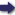(London :  Kegan Paul, Trench, Trübner & Co.,  1910.)

 Tools

## Search this bookPrev Page 54 Next``` 54 ALBERUNTS INDIA. add to this number the months which have elapsed between that ist Sha'ban and the ist Eabi' I. of the gauge-year, we get the sum of 3571, and, together with the adhimdsa months, 3680 months, i.e. 110,400 days. The corresponding number of unardtra days is 1727, and a remainder of 319 avamas. Subtracting these days, we get the remainder 108,673. If we now sub¬ tract I and divide the remainder by 7, the computation is correct, for the remainder is 4, i.e. the day of the gauge-date is a Wednesday, as has above (p. 48) been stated. Method of The method of Durlabha, a native of Multan, is the Durlabha of . Multan. following :—He takes 848 years and adds thereto the Laukika-kala. The sum is the Sakakala. He subtracts therefrom 854, and changes the remainder of years into months. He writes them down together with the past months of the current year in three different places. The lower number he multiplies by y/, and divides the product by 69,120. The quotient he subtracts from the middle number, doubles the remainder, and adds thereto 29. The sum he divides by 65, so as to get adhimdsct months. He adds them to the upper number and multiplies the sum by 30. He writes down the product together with the past days of the current month in two different places. He multiplies the lower number by 11 and adds to the product 686. The sum he writes underneath. He divides it by 403,963, and adds the quotient to the middle number. He divides the sum by 703. The quotient represents the unctrdtra days. He subtracts them from the upper number. The remainder is the civil aharganct, i.e. the sum of the civil days of the date in question. We have already in a former place mentioned the outlines of this method. After the author, Durlabha, had adopted it for a particular date, he made some additions, whilst the bulk of it is unchanged. How¬ ever, the Karanasara forbids introducing any innovations ■ ```Prev Page 54 Next